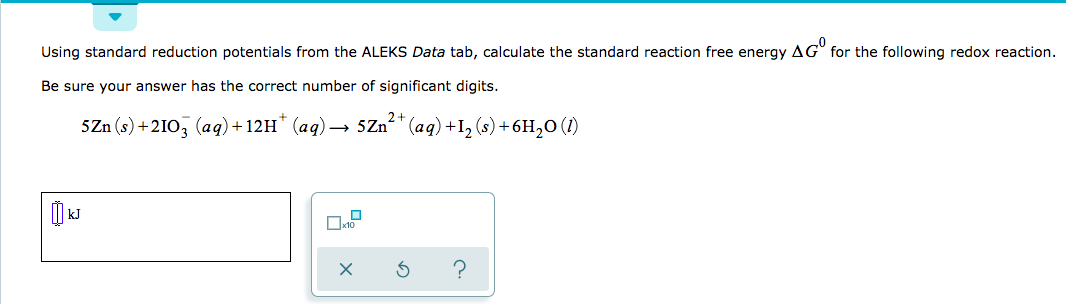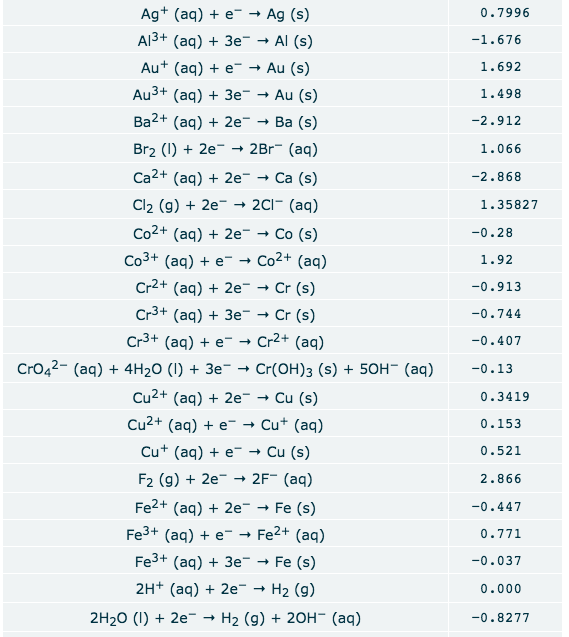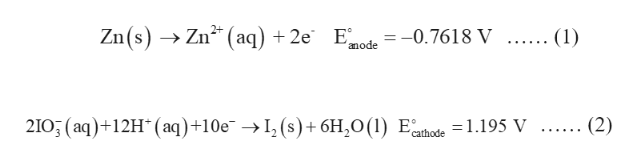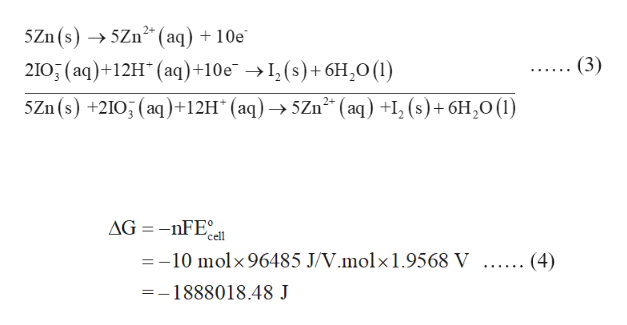# Using standard reduction potentials from the ALEKS Data tab, calculate the standard reaction free energy AG for the following redox reaction.Be sure your answer has the correct number of significant digits.2+SZn (s)+2103 (aq)+12H (aq)- 5Zn" (aq) +12 (s)+6H20 (I)to? Ag (aq)e- Ag (s)Al3+ (aq) 3e Al (s)0.7996-1.676Au (aq) e" Au (s)1.692Au3+ (aq) 3eAu (s)1.498Ba2+ (aq)2eBa (s)-2.912Br2 ()2e - 2Br (aq)1.066Ca2+ (aq)2e -- Са (s)-2.868Cl2 (g)2e » 2Cl (aq)1.35827Co2+ (aq) 2eCo (s)-0.28Co3+ (aq) e- Co2+ (aq)1.92Cr2+ (aq) 2eCr (s)-0.913Cr3+ (ag) 3e Cr (s)-0.744Cr3+ (aq) e- Cr2+ (aq)-0.407CrO42- (aq) 4H20 (I) +3eCr(OH)3 (s)50H- (aq)-0.13Cu2+ (aq)2e-Cu (s)0.3419Cu2+ (aq) e Cu (aq)0.153Cu (aq)e-Cu (s)0.521F2 (g)2e 2F (aq)Fe2+ (aq)2e2.866Fe (s)-0.447Fe3+ (aq)e"- Fe2+ (aq)0.771Fe3+ (aq) 3eFe (s)-0.0372H+ (aq) 2eH2 (g)0.0002H20 ()2e- H2 (g) + 20H (aq)-0.8277

Question
38 views

If you need the rest of the graph let me knowhelp_outlineImage TranscriptioncloseUsing standard reduction potentials from the ALEKS Data tab, calculate the standard reaction free energy AG for the following redox reaction. Be sure your answer has the correct number of significant digits. 2+ SZn (s)+2103 (aq)+12H (aq)- 5Zn" (aq) +12 (s)+6H20 (I) to ? fullscreenhelp_outlineImage TranscriptioncloseAg (aq)e- Ag (s) Al3+ (aq) 3e Al (s) 0.7996 -1.676 Au (aq) e" Au (s) 1.692 Au3+ (aq) 3e Au (s) 1.498 Ba2+ (aq)2e Ba (s) -2.912 Br2 ()2e - 2Br (aq) 1.066 Ca2+ (aq)2e - - Са (s) -2.868 Cl2 (g)2e » 2Cl (aq) 1.35827 Co2+ (aq) 2e Co (s) -0.28 Co3+ (aq) e- Co2+ (aq) 1.92 Cr2+ (aq) 2e Cr (s) -0.913 Cr3+ (ag) 3e Cr (s) -0.744 Cr3+ (aq) e- Cr2+ (aq) -0.407 CrO42- (aq) 4H20 (I) +3e Cr(OH)3 (s) 50H- (aq) -0.13 Cu2+ (aq)2e- Cu (s) 0.3419 Cu2+ (aq) e Cu (aq) 0.153 Cu (aq)e-Cu (s) 0.521 F2 (g)2e 2F (aq) Fe2+ (aq)2e 2.866 Fe (s) -0.447 Fe3+ (aq)e"- Fe2+ (aq) 0.771 Fe3+ (aq) 3e Fe (s) -0.037 2H+ (aq) 2e H2 (g) 0.000 2H20 ()2e- H2 (g) + 20H (aq) -0.8277 fullscreen
check_circle

Step 1

The reaction is written as follows:

5Zn(s) + 2IO3- (aq) + 12H+(aq) →5Zn2+(aq) + I2(s) + 6H2O(l)

The oxidation half reaction is shown in equation (1).

The reduction half reaction is shown in equation (2).help_outlineImage TranscriptioncloseZn(s) Zn2 (aq) 2e E^ -0.7618 V ...... (1) anode 210, (aq)12H (aq) +10e ->I, (s)+6H20(1) Ehode 1.195 V ...... (2) fullscreen
Step 2

The standard cell potential is calculated as follows:

Step 3

The overall reaction is written by combining equation (1) and (2) and multiplying equation (1) by ...help_outlineImage Transcriptionclose5Zn (s)>5Zn2* (aq) + 10e (3) 210, (aq)+12H (aq)+10e -I, (s)+6H20(1) SZn(s) +210 (aq)+12H* (aq) -> 5Zn2 (aq) +I, (s)+6H20(1) AG=-nFEl =-10 molx 96485 J/V.molx1.9568 V ...... (4) -1888018.48 J fullscreen

### Want to see the full answer?

See Solution

#### Want to see this answer and more?

Solutions are written by subject experts who are available 24/7. Questions are typically answered within 1 hour.*

See Solution
*Response times may vary by subject and question.
Tagged in

### Chemistry